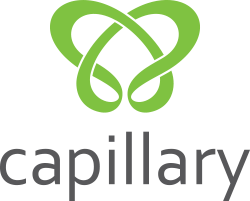Array Modification
Tag(s):

## Algorithms, Easy, Greedy algorithm

Problem
Editorial
Analytics

You are given an array $A$ of $N$ integers. For every index $i$ of the array $A$, you need to select an index $j$  such that $j \neq i$ and the product of $A[i]$ and $A[j]$ is maximum for that $i$ . Finally, you need to print the sum of $A[i] * A[j]$ for all the indices $i$ . Since this sum can be large, you need to print it to the modulo $10^9 + 7$.
Note: Please refer to the sample explanation section.

Input format

• First line: Integer $N$ denoting the number of elements in the array $A$
• Next line: $N$ space-separated integers denoting the elements of the array $A$

Output format

Print the required sum to the modulo $10^9+7$

Constraints
$2 \le N \le 10^5$

$-10^{18} \le A[i] \le 10^{18}$

SAMPLE INPUT
4
1 -9 2 -1
SAMPLE OUTPUT
22
Explanation

For an element with the index 1, you can choose another index as 3, so its maximum product will be $1 * 2 = 2$
For an element with the index 2, you can choose another index as 4, so its maximum product will be $-9 * -1 = 9$
For an element with the index 3, you can choose another index as 1, so its maximum product will be $2 * 1 = 2$
For an element with the index 4, you can choose another index as 2, so its maximum product will be $-1 * -9 = 9$
Hence, the final answer will be $2 + 9 + 2 + 9 = 22$.

Time Limit: 2.0 sec(s) for each input file.
Memory Limit: 256 MB
Source Limit: 1024 KB
Marking Scheme: Marks are awarded when all the testcases pass.
Allowed Languages: Bash, C, C++, C++14, Clojure, C#, D, Erlang, F#, Go, Groovy, Haskell, Java, Java 8, JavaScript(Rhino), JavaScript(Node.js), Julia, Kotlin, Lisp, Lisp (SBCL), Lua, Objective-C, OCaml, Octave, Pascal, Perl, PHP, Python, Python 3, R(RScript), Racket, Ruby, Rust, Scala, Swift, Swift-4.1, TypeScript, Visual Basic

## CODE EDITOR

Initializing Code Editor...

## This Problem was Asked inChallenge Name

Capillary .Net Hiring Challenge - February 2019

OTHER PROBLEMS OF THIS CHALLENGE
• Data Structures > Arrays